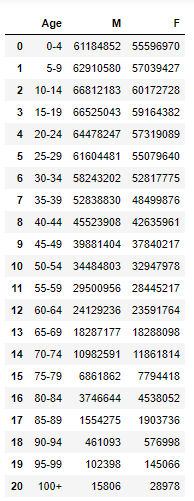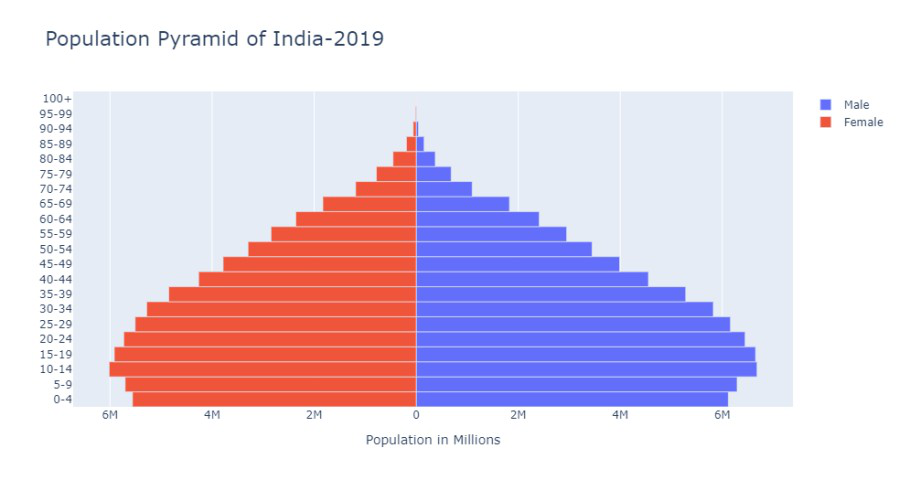Related Articles

# How to Create a Population Pyramid Using Plotly in Python?

• Last Updated : 24 Feb, 2021

A population pyramid is a graphical representation of data that contains two entities, namely the age and gender of a specific population. It is generally used by demographers to study the population. The age value is divided into sub-categories and the gender column contains the population of that specific gender who belong to this age group.

It is called the population pyramid because of its graphical shape that resembles a pyramid. In which the people belonging to the youngest age-group is kept at the bottom and the oldest at the top of the graph.

In this article, we will be studying how can we create a population pyramid in Python. To achieve our purpose we will use two additional libraries Pandas and Plotly to plot our graph. If you don’t have these libraries installed, you can install them via pip commands.

`pip install plotly`

Let’s check out how can we can make the population pyramid using the Plotly library in Python

Step 1: We will start with firstly importing the libraries in our code.

## Python

 `# Importing libraries``import` `pandas as pd``import` `plotly.graph_objects as gp`

Step 2: Reading the CSV file which contains our data.

## Python

 `data ``=` `pd.read_csv(``'India-2019.csv'``)``display(data)`

Output:Our dataset

We can notice our dataset contains three columns, the first column is ‘Age’ that contains different age range, the second and third column contains the number of people belonging to these age groups both in the male and female gender category.

Step 3: Data Preparation

On the y-axis, we will be plotting the age and on the x-axis, we will be plotting Male and Female numbers

## Python

 `y_age ``=` `data[``'Age'``]``x_M ``=` `data[``'M'``]``x_F ``=` `data[``'F'``] ``*` `-``1`

Step 4: Plotting the graph

We will create an instance of our already imported graph_object module and using this instance, we will add male and female data onto the graph one by one using the add_trace() method.

We can even customize the plot layout as per our requirements if you notice I have placed a title for my graph and set the bargap to 0, so that our bars do not have any spacing. For our values on X-axis, we can customize the graph as well like setting title on the x-axis and the tick values.

## Python

 `# Creating instance of the figure``fig ``=` `gp.Figure()`` ` `# Adding Male data to the figure``fig.add_trace(gp.Bar(y``=` `y_age, x ``=` `x_M, ``                     ``name ``=` `'Male'``, ``                     ``orientation ``=` `'h'``))`` ` `# Adding Female data to the figure``fig.add_trace(gp.Bar(y ``=` `y_age, x ``=` `x_F,``                     ``name ``=` `'Female'``, orientation ``=` `'h'``))`` ` `# Updating the layout for our graph``fig.update_layout(title ``=` `'Population Pyramid of India-2019'``,``                 ``title_font_size ``=` `22``, barmode ``=` `'relative'``,``                 ``bargap ``=` `0.0``, bargroupgap ``=` `0``,``                 ``xaxis ``=` `dict``(tickvals ``=` `[``-``60000000``, ``-``40000000``, ``-``20000000``,``                                          ``0``, ``20000000``, ``40000000``, ``60000000``],``                               ` `                              ``ticktext ``=` `[``'6M'``, ``'4M'``, ``'2M'``, ``'0'``, ``                                          ``'2M'``, ``'4M'``, ``'6M'``],``                               ` `                              ``title ``=` `'Population in Millions'``,``                              ``title_font_size ``=` `14``)``                 ``)`` ` `fig.show()`

Output:Population pyramid of India in 2019

Attention geek! Strengthen your foundations with the Python Programming Foundation Course and learn the basics.

To begin with, your interview preparations Enhance your Data Structures concepts with the Python DS Course. And to begin with your Machine Learning Journey, join the Machine Learning – Basic Level Course

My Personal Notes arrow_drop_up